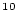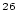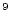Next: Converting the Be/Be ratio Up: Converting Al and Be Previous: Converting Al and Be

# Introduction

This document describes how to reduce a Be or Al isotope ratio measurement, provided by an accelerator-mass-spectrometer measurement, to aBe orAl concentration in quartz, which is the quantity needed to calculate an exposure age or an erosion rate. This process also incorporates theBe process blank and its uncertainty into the final estimate of the nuclide concentration in the sample.

The information needed to do this is as follows. For aBe measurement, one needs: 1) the measuredBe/Be isotope ratio and its 1-standard-error uncertainty; 2) the mass of Be added as carrier in the chemical processing and its uncertainty; 3) the mass of the quartz sample itself; and 4) the typical number of atoms ofBe in a laboratory process blank, and its uncertainty.

To determine this last item, the `typical' number ofBe atoms in the process blank, one also needs the measured isotope ratios and the carrier concentrations used for one or more process blanks that were run together with samples. Basically, the procedure is to first calculate the number ofBe atoms in your process blanks, then use this information to decide on the correct blank value to use in computing theBe concentrations in the samples. The discussion below goes in the opposite order - beginning with the situation for samples and then going on to a discussion of blanks - for better clarity in the explanation.

For anAl measurement, one needs the corresponding information for Al, except that Al carrier is not commonly used forAl measurements. The total amount of natural Al measured in the sample is used instead.Next: Converting the Be/Be ratio Up: Converting Al and Be Previous: Converting Al and Be
2006-05-08